## Perimeter and Area of Rectangle – Definition, Formula, Solved Examples

The Perimeter and Area of Rectangle are two important formulas in Mensuration. It calculates the space occupied by the rectangle and the length of boundaries of the rectangle. In this article, students can come across the concept of area and perimeter of rectangle deeply.

A rectangle is a quadrilateral with two equal sides and two parallel lines and four right angles. The concept of area and perimeter of rectangle formulas are explained with examples. Some of the examples of different shapes are given below.

## What is Perimeter and Area of a Rectangle?

Perimeter: Perimeter of the rectangle is the sum of all the sides of the rectangle. The rectangle has two lengths and two breadths. To find the perimeter of the rectangle we have to add the length and breadth. It is measured in units. It is denoted by P.

Area: The area of the rectangle formula helps to calculate the length and breadth of the two-dimensional closed figure. To find the area of the rectangle we have to multiply the length and breadth of the rectangle. It is measured in square units. It is denoted by A.

### Properties of Rectangle

• A Rectangle has two equal sides
• The rectangle is a quadrilateral
• The diagonals of the rectangle have the same length.
• The diagonal of the rectangle bisect each other.
• Sum of all the four angles is 360º
• Each angle of the rectangle is 90º
• If the sides of the rectangle are l and b then the diagonal of the rectangle d = √l² + b²
• The opposite sides of the rectangle are parallel.

### Derivation of Perimeter of a Rectangle

The perimeter of the rectangle is the sum of all four sides.
P = l + l + b + b
P = 2l + 2b
P = 2(l + b)
Thus the perimeter of the rectangle is = 2(l + b)

### Area of the Rectangle

The area of the rectangle is the product of length and breadth.
A = l × b

### Perimeter and Area of Rectangle Formula

• Area of Rectangle = l × b
• Perimeter of Rectangle = 2(l + b)
• Length of the rectangle = A/b
• The breadth of the Rectangle = A/l
• Diagonal of the Rectangle = √l² + b²

### Solved Problems on Area and Perimeter of a Rectangle

The formula of Perimeter and Area of Rectangle is explained step by step here with examples. Go through the below questions and solve the problems using the Area and Perimeter of the Rectangle formula.

1. Find the length of the rectangular plot whose breadth is 11 cm and the area is 165 cm². Also, find the perimeter of the rectangle?

Solution:

Given,
Area = 165 cm²
Area of the rectangle = l × b
165 sq. cm = l × 11 cm
l = 165/11
l = 15 cm
Thus the length of the rectangle is 15 cm.
We know that,
Perimeter of the rectangle = 2(l + b)
P = 2(15 + 11)
P = 2(26)
P = 52 cm
Thus the perimeter of the rectangle is 52 cm.

2. Find the Perimeter of the Rectangle whose length is 10 cm and breadth is 8 cm?

Solution:

Given, Length = 10 cm
We know that,
Perimeter of the rectangle = 2(l + b)
P = 2(10 cm + 8 cm)
P = 2(18 cm)
P = 36 cm
Therefore the perimeter of the rectangle is 36 cm.

3. Find the area of the rectangle whose length is 14 meter and width is 10 meters?

Solution:

Given,
Length = 14 meter
Width = 10 meter
We know that,
Area of the rectangle = l × w
A = 14 m × 10 m
A = 140 sq. meters
Therefore the area of the rectangle is 140 square meters.

4. A rectangular plot has its length of 16 cm and a perimeter of 60 cm. Find the width of the rectangular plot?

Solution:

Given,
Length = 16 cm
Perimeter = 60 cm
Width = ?
We know that
The perimeter of the rectangle = 2(l + w)
60 cm = 2(16 cm + w)
16 + w = 60/2
16 + w = 30
w = 30 – 16
w = 14 cm
Thus the width of the rectangular plot is 14 cm.

5. Find the area and perimeter of the rectangle whose length and breadth are 12 m and 6 m?

Solution:
Given,
length = 12 m
We know that,
Area of the rectangle = l × b
A = 12 m × 6 m
A = 72 sq. meters
Now find the perimeter of the rectangle
P = l + l + b + b
P = 12 m + 12 m + 6 m + 6 m
P = 24 m + 12 m
P = 36 m
Therefore the area and perimeter of the rectangle is 72 square meters and 36 meters.

### FAQs on Area and Perimeter of Rectangle

1. How to find the perimeter of a rectangle?

The perimeter of the rectangle can be calculated by adding all the sides of the rectangle.

2. What is the area of the rectangle?

The area of the rectangle is defined as the space occupied by the rectangle or closed figure. It is the product of length and breadth.

3. What is the unit for the perimeter of a rectangle?

The unit for the perimeter of the rectangle is cm or meters.

## Area and Perimeter Definition, Formulas | How to find Area and Perimeter?

Area and Perimeter is an important and basic topic in the Mensuration of 2-D or Planar Figures. The area is used to measure the space occupied by the planar figures. The perimeter is used to measure the boundaries of the closed figures. In Mathematics, these are two major formulas to solve the problems in the 2-dimensional shapes.

Each and every shape has two properties that are Area and Perimeter. Students can find the area and perimeter of different shapes like Circle, Rectangle, Square, Parallelogram, Rhombus, Trapezium, Quadrilateral, Pentagon, Hexagon, and Octagon. The properties of the figures will vary based on their structures, angles, and size. Scroll down this page to learn deeply about the area and perimeter of all the two-dimensional shapes.

## Area and Perimeter Definition

Area: Area is defined as the measure of the space enclosed by the planar figure or shape. The Units to measure the area of the closed figure is square centimeters or meters.

Perimeter: Perimeter is defined as the measure of the length of the boundary of the two-dimensional planar figure. The units to measure the perimeter of the closed figures is centimeters or meters.

### Formulas for Area and Perimeter of 2-D Shapes

1. Area and Perimeter of Rectangle:

• Area = l × b
• Perimeter = 2 (l + b)
• Diagnol = √l² + b²

Where, l = length

2. Area and Perimeter of Square:

• Area = s × s
• Perimeter = 4s

Where s = side of the square

3. Area and Perimeter of Parallelogram:

• Area = bh
• Perimeter = 2( b + h)

Where, b = base
h = height

4. Area and Perimeter of Trapezoid:

• Area = 1/2 × h (a + b)
• Perimeter = a + b + c + d

Where, a, b, c, d are the sides of the trapezoid
h is the height of the trapezoid

5. Area and Perimeter of Triangle:

• Area = 1/2 × b × h
• Perimeter = a + b + c

Where, b = base
h = height
a, b, c are the sides of the triangle

6. Area and Perimeter of Pentagon:

• Area = (5/2) s × a
• Perimeter = 5s

Where s is the side of the pentagon
a is the length

7. Area and Perimeter of Hexagon:

• Area = 1/2 × P × a
• Perimeter = s + s + s + s + s + s = 6s

Where s is the side of the hexagon.

8. Area and Perimeter of Rhombus:

• Area = 1/2 (d1 + d2)
• Perimeter = 4a

Where d1 and d2 are the diagonals of the rhombus
a is the side of the rhombus

9. Area and Perimeter of Circle:

• Area = Πr²
• Circumference of the circle = 2Πr

Where r is the radius of the circle
Π = 3.14 or 22/7

10. Area and Perimeter of Octagon:

• Area = 2(1 + √2) s²
• Perimeter = 8s

Where s is the side of the octagon.

### Solved Examples on Area and Perimeter

Here are some of the examples of the area and perimeter of the geometric figures. Students can easily understand the concept of the area and perimeter with the help of these problems.

1. Find the area and perimeter of the rectangle whose length is 8m and breadth is 4m?

Solution:

Given,
l = 8m
b = 4m
Area of the rectangle = l × b
A = 8m × 4m
A = 32 sq. meters
The perimeter of the rectangle = 2(l + b)
P = 2(8m + 4m)
P = 2(12m)
P = 24 meters
Therefore the area and perimeter of the rectangle is 32 sq. m and 24 meters.

2. Calculate the area of the rhombus whose diagonals are 6 cm and 5 cm?

Solution:

Given,
d1 = 6cm
d2 = 5 cm
Area = 1/2 (d1 + d2)
A = 1/2 (6 cm + 5cm)
A = 1/2 × 11 cm
A = 5.5 sq. cm
Thus the area of the rhombus is 5.5 sq. cm

3. Find the area of the triangle whose base and height are 11 cm and 7 cm?

Solution:

Given,
Base = 11 cm
Height = 7 cm
We know that
Area of the triangle = 1/2 × b × h
A = 1/2 × 11 cm × 7 cm
A = 1/2 × 77 sq. cm
A = 38.5 sq. cm
Thus the area of the triangle is 38.5 sq. cm.

4. Find the area of the circle whose radius is 7 cm?

Solution:

Given,
We know that,
Area of the circle = Πr²
Π = 3.14
A = 3.14 × 7 cm × 7 cm
A = 3.14 × 49 sq. cm
A = 153.86 sq. cm
Therefore the area of the circle is 153.86 sq. cm.

5. Find the area of the trapezoid if the length, breadth, and height is 8 cm, 4 cm, and 5 cm?

Solution:

Given,
a = 8 cm
b = 4 cm
h = 5 cm
We know that,
Area of the trapezoid = 1/2 × h(a + b)
A = 1/2 × (8 + 4)5
A = 1/2 × 12 × 5
A = 6 cm× 5 cm
A = 30 sq. cm
Therefore the area of the trapezoid is 30 sq. cm.

6. Find the perimeter of the pentagon whose side is 5 meters?

Solution:

Given that,
Side = 5 m
The perimeter of the pentagon = 5s
P = 5 × 5 m
P = 25 meters
Therefore the perimeter of the pentagon is 25 meters.

### FAQs on Area and Perimeter

1. How does Perimeter relate to Area?

The perimeter is the boundary of the closed figure whereas the area is the space occupied by the planar.

2. How to calculate the perimeter?

The perimeter can be calculated by adding the lengths of all the sides of the figure.

3. What is the formula for perimeter?

The formula for perimeter is the sum of all the sides.

## Absolute Value of an Integer Definition, Examples | How to find the Absolute Value of an Integer?

Wanna learn all the basics of Integers concepts? Then start with the absolute value of an integer. Integer Absolute Value is an important and basic concept to learn other concepts of Integers. Refer to all the rules, definitions, solved examples, types, etc to understand the concept more clearly. Follow the below sections to get more idea on how to find the Absolute Value of an Integer in detail and Solved Problems for finding the Integer Absolute Value using different approaches.

## Absolute Value of an Integer – Introduction

Integers Absolute Value is the distance of that integer value from zero irrespective of (positive or negative) direction. While considering the absolute value, its numerical value is taken without taking the sign into consideration.

An integer is any positive or negative whole number. Therefore, the positive integer has a negative sign and vice versa.

A positive number is a number that is greater than zero. It is represented by a “+” symbol and can be written with or without a symbol in front of it. The gain in some value or something is written with a positive number. A negative number is a number that is less than zero. It is represented by a “-” symbol and can be written with a “-” symbol in front of it. The loss in some value or something is written with a negative number.

A number line is a kind of diagram on which the numbers are marked at intervals. These are used to illustrate simple and easy numerical operations. Using the number line allows seeing a number is in relationship to other numbers and from zero. Zero, is present in the middle and separates positive and negative numbers. On the right side of zero, we can find numbers that are greater than zero (positive numbers) and on the left side, we can find numbers that are less than zero (negative numbers). The absolute value of the integer is the same as the distance from zero to a specific number.

### Representation of Absolute Value of an Integer

Integer Absolute Value is represented with two vertical lines. i.e., | |, one on either side of the integer.

|a| = a, when a is the positive integer

|a| = -a, when a is the negative integer

Examples:

1. Absolute integer value of -15 is written as |-15| = 15 {here mod of -15 = 15}
2. Absolute integer value of 8 is written as |8| = 8 {here mod of 8 = 8}

### Adding and Subtracting Absolute Value of Integers

To add 2 integers with the same (positive or negative) sign, add absolute values and assign the sum with the same sign as same both values.

Example:

(-7) + (-4) = -(7 + 4)= – 11.

To add 2 integers with different signs, find the difference in absolute integer values and give that product the same sign of the largest absolute value.

Example:

(-7)+(2)= -5

How to find the absolute value?

1. First of all, find the absolute values of (7 and 2)
2. Find the difference of numbers between 7 and 2 (7-2=5)
3. Find the sign of the largest absolute value i.e., negative, as 7 is of (-)sign
4. Add the sign to the difference we got in Step 2.
5. Hence the final solution is -5

When an integer is subtracted or added by another integer, the result will be an integer.

### Multiplication of Absolute Value of Integers

To multiply the integer values, we have to multiply the absolute values. If the integers that are to be multiplied have the same sign, then the result will be positive. If both the integers have different signs, then the result will be negative.

When an integer is multiplied by another integer, then the result will be an integer itself.

### Absolute Value of Integers Examples

Question 1:

Write the absolute value of each of the following?

(i) 15

(ii) -24

(iii) -375

(iv) 0

(v) +7

(vi) +123

Solution:

(i) 15

(ii) 24

(iii) 375

(iv) 0

(v) 7

(vi) 123

Question 2:

Evaluate the following integers

(i) |-7| + |+5| + |0|

(ii) |10| – |-15| + |+12|

(iii) – |+3| – |-3| + |-6|

(iv) |-8| – |17| + |-12|

Solution:

(i) 12

(ii) 7

(iii) 0

(iv) 3

Question 3:

State whether the statements are true or false?

(i) The absolute value of -3 is 3.

(ii) The absolute value of an integer is always greater than the integer.

(iii) |+5| = +5

(iv) |-5| = -5

(v) – |+5| = 5

(vi) – |-5| = -5

Solution:

(i) True

(ii) False

(iii) True

(iv) False

(v) False

(vi) True

### Methods to Compute the Absolute Value of Integer

There are 3 approaches to find the Integers Absolute value. These approaches are helpful while solving problems. Finding the absolute value is the first step in solving the problems.

Method 1:

As the absolute value of the integer is always positive. For the positive integer, the absolute number is the number itself. For the negative number, the absolute number is multiplied by other negative numbers.

Method 2:

Negative numbers are saved in 2’s complement form. To get the absolute number, toggle bits of the number, then 1 to the result.

Method 3:

The built-in function library finds the absolute value of an integer.

To solve most of the problems, knowing the absolute value of the integer is important. This method is used in the real world and it has all sorts of math clues. Absolute values are often used in distance problems and also sometimes used for inequalities. Integers Absolute values are really helpful to get clarity on many things.

We have mentioned the important concept of Integers Absolute Value in the above article. Hope you got a clear idea of definitions, rules, and how to solve the problems. If you have any doubts, you can contact us from the below comment box. You can also directly ping us to know other information. Stay tuned to our page to get the latest and important information about all mathematical concepts.

## Lines and Angles – Definition, Types, Properties, Solved Problems

Lines and Angles concepts are explained by experts in order to help students to get excellent marks in their examination. The line is a combination of infinite points that has both directions infinitely. There are different lines available such as intersecting lines, perpendicular lines, transversal lines, etc. An angle is nothing but a combination of two rays with a common endpoint.

Based on the operations performed on a line, then the lines are classified as Parallel Lines, Perpendicular Lines, Transversal, etc. In the same way, the angles are classified as Complementary Angles, Supplementary Angles, Adjacent Angles, Vertically Opposite Angles based on their operation.

## Quick Links of Lines and Angles

Working on Lines and Angles problems will help students to get an idea about how to solve the problems. All the concept links are given below. Just check out the concepts and open the topic that is difficult for you. After that solve all the problems available on that topic to get a grip on that entire topic.

### Line Segment

The line segment is a line that has two endpoints with a fixed length.### Ray

A ray is a line that has only one endpoint and extends infinitely in another direction. From the below figure, point A is the and point.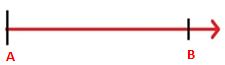### Perpendicular Lines

Two lines are said to be Perpendicular Lines when they meet at a single point and forms a right angle. From the below figure, PQ and RS are two lines and they intersect at a point x. Also, the two lines PQ and RS form a right angle 90º.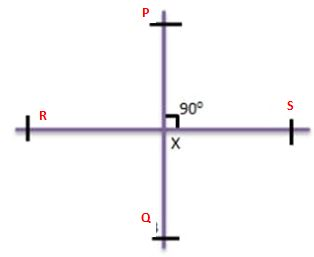### Parallel Lines

Two lines are said to be Parallel Lines when they do not intersect each other and they have the same distance throughout the plane. From the below figure, A and B are two parallel lines.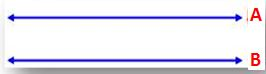### Transversal Line

A transversal Line is a line that intersects two lines at distinct points. From the below figure, C is the Transversal Line, that intersects the lines A and B at two points D and E.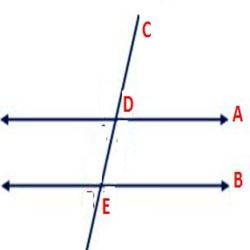### Properties of Lines

• The points those are not lie on the same line are called non-collinear points.
• The points that lie on the same line are called Collinear points

### Acute Angle

The angle that is less than the right angle is called Acute Angle.

### Obtuse Angle

The angle that is more than the right angle is called Acute Angle.

### Right Angle

The angle with 90 degrees is called as Right Angle.

### Straight Angle

The angle that is equal to 180 degrees is called Straight Angle.

### Complementary Angles

If the sum of the two angles is 90 degrees, then they are called Complementary Angles.

### Supplementary Angles

If the sum of the angles is 180 degrees, then those angles are called Supplementary Angles.

### Properties of Angles

• An angle is formed at a point where two rays intersecting.
• If the angle is more than 180 degrees and less than 360 degrees, then it is called a reflex angle.
• If the two angles are adjacent angles and add up to 180 degrees then they form linear pair.

We have given solutions for all questions with step by step explanation in understandable language. Therefore, students can get great knowledge on the entire concepts of Lines and Angles. All the questions and answers that are present in the updated syllabus are included on our website with individual concept explanation. So, students who want to learn a single topic can go through the required link available below and learn it from the concerned link.

Are you searching for Lines and Angles Material? You have free access to Lines and Angles Material on our website. You can read and practice all problems either online or offline. So, immediately bookmark our website and practice all the math problems at your comfortable times. Do hard work and get complete knowledge on the Lines and Angles concept. Clear your doubts by contacting us through the comment section.

## Triangle on Same Base and between Same Parallels Theorem – Solved Examples

When two triangles lie on the same base and between the same parallels, then those triangles measure equal area. Check the proof for Triangles on the same base and between the same parallel are equal in Area. Get the relationship between the areas of triangles having the same base in the following sections. For the reference of students, we have also included the solved examples on Triangle on the Same Base and between Same Parallels.

## Proof for Area of Triangles are Equal Having the Same Base

Get a detailed explanation for how the areas of triangles are equal when their bases are the same and between the same parallels.

In the following figure, ∆ABD and ∆DEF having equal base length and between the same parallels BF and AD.In this case, Area of △ ABC = Area of △ DEF

To prove that Triangle on the Same Base and between Same Parallels have the same area, follow these instructions.

Let us take △ ABC and △ ABD on the same base AB and between the same parallels AB and CD.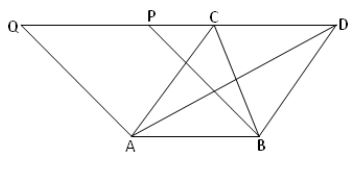Draw a parallelogram ABPQ with AB base and lying between the same parallels AB and Q.

The relationship between the area of the triangle and parallelogram says that when they lie on the same base and between the same parallels, then the triangle is half of the parallelogram area.

So, Area of ∆ ABC = 1/2 x Area of parallelogram ABPQ

In the same way, Area of ∆ ABD = 1/2 x Area of parallelogram ABPQ

Therefore, Area of ∆ ABC = Area of ∆ ABD.

Hence proved.

### Questions on Triangles on the Same Base and between Same Parallels

Example 1.

AD is the median of ∆ABC and ∆ADC. E is any point on AD. Show that area of ∆ABE = area of ∆ACE.

Solution: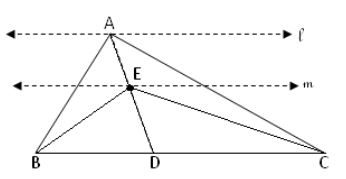Here, AD is the median for △ ABC.

So, BD = CD

In the above figure, △ ABD and △ ADC are having equal bases BD and CD and they are between the same parallels BC and U.

So, Area of △ ADC = Area of △ ABD —– (i)

Therefore, Ed is the median for △ BCE

Now, BDE and CDE are having the equal base length BD = CD and between the same paralleks BC and m, then

Area of △ CDE = Area of △ BDE —— (ii)

Subtracting equation (i) and equation (ii), we get

Area of △ ADC – Area of △ CDE = Area of ∆ABD – Area of ∆BED

Area of △ ABE = Area of △ ACE

Hence, shown.

Example 2.

Prove that the medians of the triangle divide it into two triangles having equal area?

Solution:AD is the median of the ∆ BCA and AE is the altitude of ∆ BCA and also ∆ ADC.

And AE is perpendicular to BC

Here, AD is the median of ∆ ABC

Therefore, BD = CD

On multiplying both sides of the equation by AE, we get

BD x AE = CD x AE

Again multiply both sides by 1/2

1/2 x BD x AE = 1/2 x CD x AE

Area of ∆ABD = Area of ∆ADC

Hence shown.

## Triangle and Parallelogram on Same Base and between Same Parallels | Example Questions

If a triangle and parallelogram having the same base and between the same parallels, then the area of the triangle is equal to half the area of the parallelogram. Get the example problems and solutions on Triangle and Parallelogram on the Same Base and between Same Parallels. Also, find the relationship between them in the following sections explained step by step.

## Proof for Area of Triangle Equal to Half of the Parallelogram having the Same Base

In the following figure, parallelogram ABCD and ∆ABD are on the same base AB and between the same parallels AF and DC. Get the area of the triangle ABD and area of the parallelogram ABCD and check their areas.Area of the parallelogram ABCD = base x height

= AB x CF

Here, CF and DE area parallel lines CF = DE.

Area of the parallelogram = AB x DE = AB x CF.

Area of △ ABD = 1/2 x base x height

= 1/2 x AB x DE

= 1/2 x Area of the parallelogram ABCD

Therefore, Area of the parallelogram ABCD = 1/2 x Area of △ ABD

Hence proved.

Here are various case studies for the area of the parallelogram is half of the area of the triangle when they lie on the same base.

1. When a triangle and a parallelogram lies on the same base and have the same altitude, then the area of the triangle is equal to half of the parallelogram.
2. If they lie between the same parallels and have the same altitude, then the area of the triangle is equal to half of the area of the parallelogram.
3. When the triangle and rectangle have the same base and between the same parallels, then the area of the triangle is half of the area of the rectangle.

### Example Questions for Triangle and Parallelogram on Same Base and between Same Parallels

Example 1:

∆ ABD and parallelogram ABCD are on the same base AB. If the base and altitude of the parallelogram are 10 cm and 6 cm, find the area of the triangle.

Solution:

Given that,

Base of the parallelogram = 10 cm

Altitude of the parallelogram = 6 cm

Area of the parallelogram = base x altitude

= 10 x 6 = 60 cm²

We know that when a triangle and parallelogram having the same base and between the same parallels then the area of the triangle is equal to half of the area of the parallelogram.

So, area of △ ABD = 1/2x Area of the parallelogram ABCD

= 1/2 x 60 = 30 cm²

Therefore, triangle area is 30 cm².

Example 2:

∆ ABD and parallelogram ABCD are on the same base AB. If the base and height of the triangle is 16 cm and 12 cm, find the area of the parallelogram.

Solution:

Given that,

The base length of the triangle = 16 cm

Height of the triangle = 12 cm

Area of ∆ ABD = 1/2 x base x height

= 1/2 x 16 x 12

= 1/2 x 192 = 96 cm²

We know that when a triangle and parallelogram having the same base and between the same parallels then the area of the parallelogram is equal to double the area of the parallelogram.

Area of the parallelogram ABCD = 2 x Area of ∆ ABD

= 2 x 96 = 192 cm²

Therefore, parallelogram area is 192 cm².

Example 3:

In the adjacent figure, BCDE is a rectangle and ABC is a triangle show that area of the triangle ABC is equal to half of the area of the rectangle BCDE.Solution:

Given that,

Triangle ABC and rectangle BCDE lies on the same base BC and between the same parallels BC and ED>

Area of triangle ABC = 1/2 x base x height

= 1/2 x BC x AP

AP and Cd are parallel lines and having the same length. So, AP = CD.

△ ABC area = 1/2 x BC x AP = 1/2 x BC x CD

Area of the rectangle = length x breadth

= BC x CD

= 1/2 x Area of △ ABC.

Therefore, the Area of the rectangle BCDE = 1/2 x Area of △ ABC

Hence, shown.

## Parallelograms and Rectangles on Same Base and between Same Parallels – Theorem, Examples

When one parallelogram and a rectangle lies on the same base and between the same parallels then they measure equal area. Here we are providing the example questions, answers for Parallelograms, and Rectangles on Same Base and between the Same Parallels. We are also offering proof that shows parallelogram and rectangle on same between same parallels have the same area.

## Proof for Rectangles and Parallelograms on the Same Base have Equal Area

Below-mentioned is the steps for the parallelogram and rectangles having the same base and between the same parallels having equal area.

In the following figure, ABCD is a parallelogram and ABEF is the rectangle that lies on the same base AB and the same parallels AB and DF.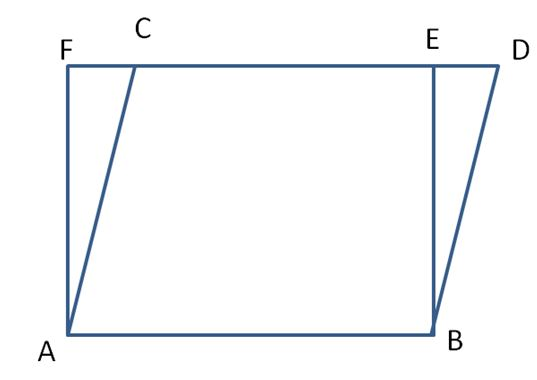Area of parallelogram ABCD = base x altitude

AF is the altitude of the parallelogram.

So, Parallelogram area = AB x AF.

Area of the rectangle ABEF = base x height

= AB x AF

Therefore, the area of the parallelogram ABCD = Area of the rectangle ABEF.

### Solved Examples for Parallelograms and Rectangles on Same Base and between Same Parallels

Example 1:

Parallelogram ABCD and rectangle ABFE have the same AB and the length and breadth of the rectangle are 15 cm and 9 cm. Find the area of the parallelogram.

Solution:

Given that,

Length of the rectangle l = 15 cm

The breadth of the rectangle b = 9 cm

Parallelogram ABCD and rectangle ABFE have the same AB.

So, area of the parallelogram = Area of the rectangle.

Area of the rectangle = length x breadth

= 15 x 9 = 135 cm²

Therefore, area of the parallelogram is 135 cm².

Example 2:

In the adjacent figure, ABCD is a parallelogram and EFCD is a rectangle. Also, AL ⊥ DC. Prove that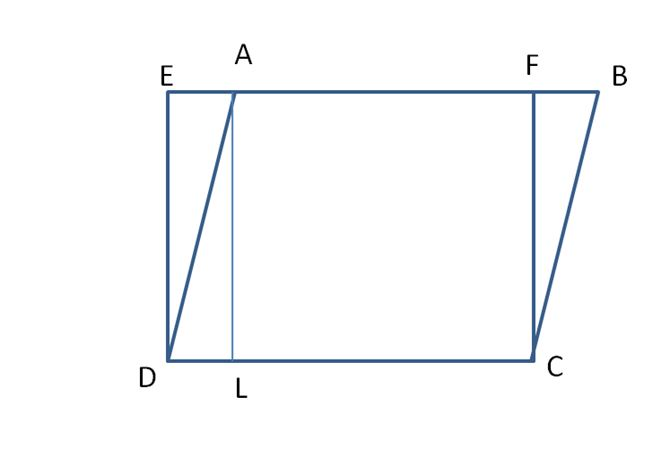(a) Area (ABCD) = Area (EFCD)

(b) Area (ABCD) = DC × AL

Solution:

(a)

Rectangle is CDEF.

Parallelogram is ABCD.

In the given figure, AL and DE are parallel and have the same length. So, DE = AL.

Area of ABCD = CD x AL

= CD x DE square units.

Area of EFCD = CD x DE

So, Area of EFCD = Area of ABCD

Hence proved.

(b)

As ABCD is a parallelogram.

Area of ABCD = base x altitude

The base is CD and the altitude is AL.

Therefore, Area of ABCD = CD x AL

Hence proved.

Example 3:

Parallelogram ABCD and rectangle ABFE have the same AB and the base and height of the parallelogram are 28 cm and 16 cm. Find the area of the rectangle.

Solution:

Given that,

Base of the parallelogram = 28 cm

Height of the parallelogram = 16 cm

Area of the parallelogram = base x height

= 28 x 16 = 448 cm²

We know, that when Parallelograms and Rectangles on Same Base and between Same Parallels, then the area of the parallelogram = area of the rectangle.

So, the area of the rectangle = 448 cm².

## Parallelograms on the Same Base and between Same Parallels Theorem, Example Questions

Students can check how parallelograms having the same base and between the same parallels are equal in area and proof for that. When two or more parallelogram lies on the same base and between same parallels then they have the same area. Also, find the solved example questions here explaining the concept and learn the concept behind them.

## Proof for Theorem: Parallelogram on the Same Base & Between Same Parallels are Equal in Area

Let us draw a parallelogram ABCD on a cardboard sheet or thick sheet of paper.Now, draw a line segment DE as shown in the figure.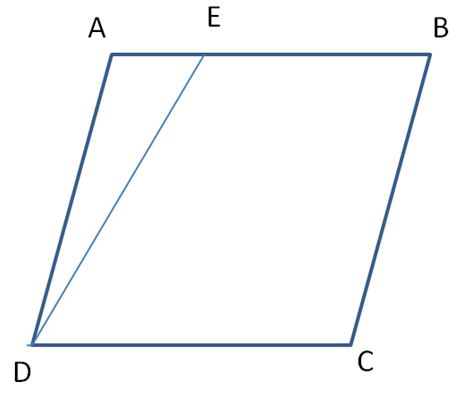Now, cut the triangle ADE congruent to triangle ADE in a seperate sheet with the help of a tracing paper and place the triangle ADE in such a way that AD coincides BC. The entire process is as shown below.Note that there are two parallelograms ABCD and EE’CD on the same base CD and between the same parallel AE’ and CD.

∆ADE ≅ ∆ A’ D’ E’

Therefore, Area of ADE = Area of A’D’E’

Also, Area of ABCD = Area of ADE + Area of EBCE

= Area of A’D’E’ + Area of EBCD

= Area of EE’CD

So, the two parallelograms are in an equal area.

### Parallelograms on Same Base and Between Same Parallels Examples

Example 1:

A farmer was having a field in the form of a parallelogram ABCD. She took any point P on AB and joined it to points C and D. In how many parts the fields are divided? What are the shapes of these parts? The farmer wants to sow wheat and pulses in equal portions of the field separately. How should she do it?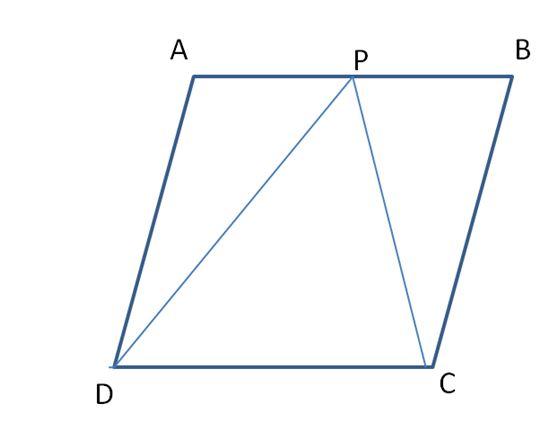Solution:

If DP and CP are joined parallelogram ABCD is divided into three triangles.

They are △ APD, △ CDP, △ BCP

△ CDP and parallelogram ABCD are on the same base i.e CD and between parallel sides CD ∥ AC

∴ Area of △ CDP = 1/2 x area of parallelogram ABCD —- (i)

∴ Area of △ APD + Area of △ BCP = 1/2 x area of parallelogram ABCD —- (ii)

From equation (i) and equation (ii)

Area of △ CDP = Area of △ APD + Area of △ BCP

∴ The farmer can use the part of the field to sow wheat, i.e. Area of △ APD + Area of △ BCP, and in the same area he can use the Area of △ CDP to sow pulses.

Example 2:

Parallelograms ABCD and ABEF are situated on the opposite sides of AB in such a way that D, A, F are not collinear. Prove that DCEF is a parallelogram, and parallelogram ABCD + parallelogram ABEF = parallelogram DCEF.

Solution: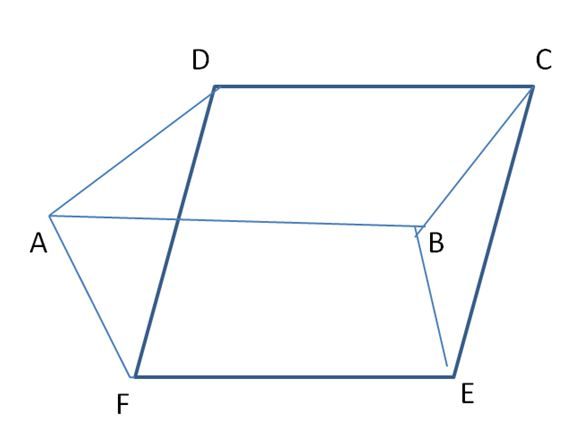AB and DC are two opposite sides of parallelogram ABCD,

Therefore, AB ∥ DC and AB = DC

Again, AB and EF are two opposite sides of parallelogram ABEF

Therefore, AB ∥ EF and AB ∥ EF

Therefore, DC ∥ EF and DC = EF

Therefore, DCEF is a parallelogram.

Therefore, ∆ADF and ∆BCE, we get

AD = BC (opposite sides of parallelogram ABCD)

AF = BE (opposite sides of parallelogram ABEF)

And DF = CE (opposite sides of parallelogram CDEF)

Therefore, ∆ADF ≅ ∆BCE (side – side – side)

Therefore, polygon AFECD – ∆BCE = polygon AFCED – ∆ADF

Parallelogram ABCD + Parallelogram ABEF = Parallelogram DCEF

## Figures on the Same Base and between Same Parallels | How to Identify Figures on the Same Base and between Same Parallels?

Two geometric shapes are said to be on the same base and between the same parallels, if they have a common side called the base and vertices opposite to the common base on the line parallel to the base. In the below sections, you can learn about the How to Identify figure on the same base and between the same parallels. We know the measure of the plane region enclosed by a closed figure called the area.

The area is measured in cm², m², and other square units. We also know how to calculate the area of different figures using various formulas. Here you will use the formulas to study the relationship between the area of figures when they lie on the same base and between the same parallels.

## Some Geometric Figures on the Same Base and between Same Parallels

Below-mentioned is the geometric figures for which you can change the same base and between the same parallels.

The common base for triangles ABC, BCD is BC. And both triangles lie on the same base.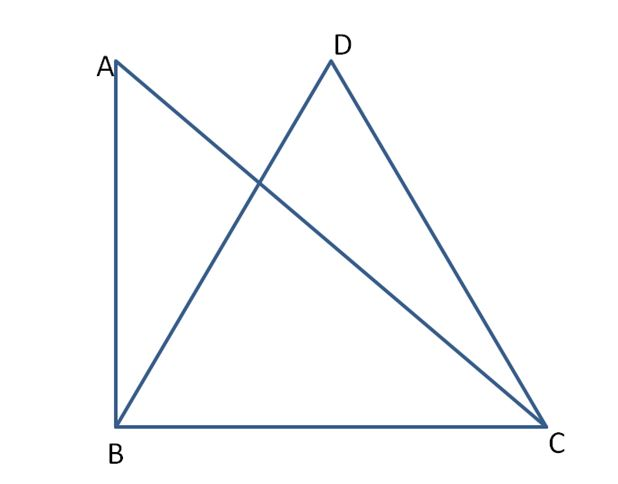Parallelograms ABCD and triangle CDE lie on the same base CD.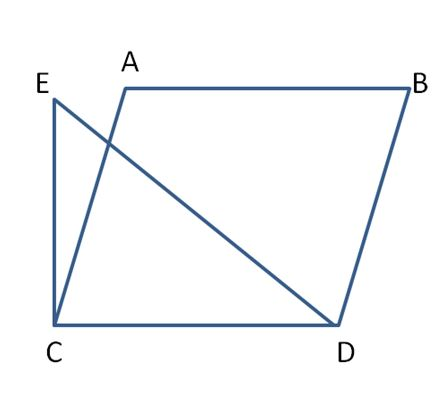Parallelograms ABCD and EFCD are on the same base DC.Trapezium ABCD and parallelogram EFCD have a common side DC. We say that trapezium ABCD and parallelogram EFCD are on the same base DC.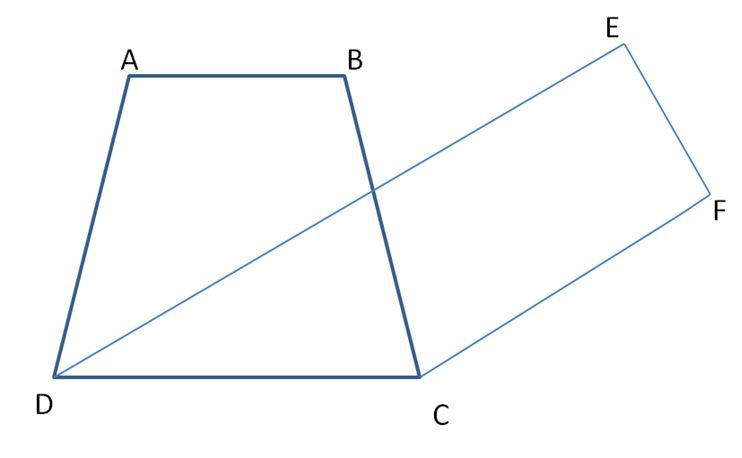### Example Questions on Figures that Lie on the Same Base and Between Same Parallels

Example 1.

ABC is right-angled at B with BC = 10 cm and AC = 15 cm. also ∆ABC and ∆BCD are on the same base BC. Find the area of ∆BCD.Solution:

In right angled ∆ ABC, AC = 15 cm and BC = 10 cm. using Pythagoras theorem, we get

AC² = AB² + BC²

15² = x² + 10²

x² = 15² – 10²

x² = 225 – 100

x² = 125

x = √125

x = 11.18 cm

Now, since ∆ ABC and ∆BCD are on the same base BC.

Therefore, area of ∆ ABC = Area of ∆BCD

1/2 × base × height = Area of ∆BCD

1/2 × 10 × 11.18 = Area of ∆BCD

Area of ∆BCD = 5 x 11.18 = 55.9 cm²

Therefore, area of ∆BCD is 55.9 cm².

Example 2.

AD is the median of ∆ABC. E is any point on AD. Show that area of ∆ABE = area of ∆ACE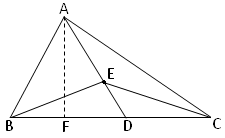Solution:

The common base for ∆ABE, ∆ACE is AE.

ABC is a triangle with AD as the median.

i.e BD = CD

E is any point on AD.

In ∆ABC,

Given that,

D is the midpoint of BC.

Since median divides the triangle into two triangles of equal area.

So, Area of ∆ABD = Area of ∆ABC —– (i)

In ∆EBC

Given that, D is the midpoint of BC.

∴ ED is median.

Since median divides the triangle into two triangles of equal area.

So, Area of ∆EBD = Area of ∆EDC —— (ii)

Subtracting equation (1) from equation (2)

Area of ∆ABD – Area of ∆EBD = Area of ∆ADC – Area of ∆EDC

Area of ∆ABE = Area of ∆ACE

Hence, proved.

Example 3.

Parallelogram PQRS and PQTU are on the same base PQ and between the same parallels PQ and UR. Area of parallelogram PQRS = 56 cm² and the altitude of the parallelogram PQTU = 7 cm. Find the length of the common side of two parallelograms.Solution:

Given that,

Area of parallelogram PQRS = 56 cm²

The altitude of the parallelogram PQTU = 7 cm

Area of parallelogram PQRS = base x height

56 = PQ x 7

PQ = 56/7

PQ = 8 cm

Therefore, the length of the common side of the two parallelograms is 8 cm.

## Properties of Division of Integers – Closure, Commutative

Properties of Division of Integers are here. Check all the rules, methods, and explanations of integer division properties. Follow simple tricks and tips to remember all rules of division of integer properties. With the Dividing Integers Properties, you can easily simplify and answer the series of operations. Refer to various properties of the division of integers and apply them while solving the problems. Scroll to the below sections to know more details regarding the division of integer properties.

## Properties of Division of Integers – Importance

Knowing the properties of the division of integers is important because most of the candidates often get confused between the properties of multiplication and division. Both the operations have different properties and are mandatory to follow all the rules. The properties help to solve many problems in an easy manner. To recall, integer numbers are positive or negative numbers, including zero. All the properties for multiplication, subtraction, division, and addition are applicable to integers. Integers are denoted by the letter “Z”.

### Rules for Division of Integers

Rule 1: The quotient of 2 positive(+) integers is positive(+).

Rule 2: The quotient of a positive(+) integer and a negative(-) integer is negative(-).

Rule 3: The quotient of 2 negative(-) integers is positive(+).

In brief, if the signs of the two integers are the same, then the result will be positive. If the signs of the two integers are different, then the result will be negative.

### Integers Division Properties

The division is the inverse operation of multiplication.

Let us take the example of whole numbers,

24/4 which means dividing 24 by 4 is nothing but finding an integer when multiplied with 4 that gives us 24, such integer is 6.

So, 24/6=4 and 24/4=6

Hence, for each whole-number multiplication statement, there are two division statements.

#### 1. Division of negative integer by positive integer

Whenever a negative number is divided by a positive number, the result will always be negative.

Steps:

1. Divide the given number as the whole number first.
2. Then add, minus symbol before the quotient. Thus we get the final result as a negative integer.

Example:

(-10)/(2) = (-5)

(-32)/(8) = (4)

#### 2. Division of positive integer by negative integer

Whenever a positive integer is divided by a negative integer, the result will always be negative.

Steps:

1. Divide the given number as the whole number first.
2. Then add, minus symbol before the quotient. Thus we get the final result as a negative integer.

Example:

81/(–9) = –9

60/(–10) = –6

#### 3. Divide a negative integer by a negative integer

Whenever a negative integer is divided by a negative integer, then the result will always be positive.

Steps:

1. Divide the given number as the whole number first.
2. Then add, plus symbol before the quotient. Thus we get the final result as a positive integer.

Example:

(–15)/(– 3)=5

(–21)/(– 7)=3

#### 4. Closure Under Division Property

Generally, the closure property is, if there are 2 integers, then the addition or subtraction of those integer results in an integer. But integers division does not follow closure property.

Example:

Let us consider the pair of integers.

(-12)/(-6) = 2 (Result is an integer)

(-5)/(-10) = -1/2 (Result is not an integer)

From the above examples, we conclude that integers are not closed under division.

#### 5. Commutative Property of Division

The commutative property states that swapping or changing the order of integers does not affect the final result. Integers division does not follow commutative property also.

Let us consider the pairs of integers.

(– 14)/(– 7)=2

(– 7)/(– 14)=1/2

(– 14)/(– 7)≠(– 7)/(– 14)

From the above examples, we conclude that integers are not commutative for integers.

#### 6. Division of Integer by Zero

Any integer divided by zero gives no result or meaningless result.

Example:

5 ÷ 0 = not defined

When zero is divided by an integer other than zero it results in zero.

Example:

0 ÷ 6 = 0

#### 7. Division of Integer by 1

When an integer is divided by 1, it gives the result as 1.

Example:

(– 7)/1=(– 7)

The above example shows that when a negative integer is divided by 1, it gives the same negative integer.

### Dividing Integers Properties Examples

Question 1:

Verify that a/(b+c)≠(a/b)+(a/c) when a = 8, b = – 2, c = 4.

Solution:

L.H.S=a/(b + c)

= 8/(-2+4)

= 8/2=4

R.H.S=(a/b)+(a/c)

= [8/(-2)]+(8/4)

= (-4)+2

= -2

Therefore, L.H.S≠R.H.S

Hence the above equation is verified.

Question 2:

(– 80)/(4) is not the same as 80/(–4). True/False

Solution:

(– 80)/(4)=-20

80/(–4)=-20

As (– 80)/(4) = 80/(–4), therefore the above statement is false.

Question 3:

Evaluate [(– 8)+4)]/[(–5)+1]

Solution: [(– 8)+4)]/[(–5)+1]

= (-4)/(-4)

= 1

Question 4:

Find the quotient of

(-15625)/(-125)

Solution:

From the question, (-15625)/(-125)

= (-15625/-125)

= (15625/125)

= 125

Question 5:

Find the value of [32+2*17+(-6)]/15

Solution:

[32+2*17+(-6)]/15

= [32+34+(-6)]/15

= (66 – 6) ÷ 15

= 60/15

= 4

### How to Divide Whole Numbers?

1. After dividing the first digit of the dividend by divisor, if the divisor is a larger number than the first digit of the dividend, then divide the first 2 digits of the dividend by divisor and so on.
2. Always, write the quotient above the dividend.
3. Multiply the quotient value by the divisor and write the product value under the dividend.
4. Subtract the product value from the dividend and bring down the next digit of that dividend.
5. Repeat solving from Step 1 until there are no digits left in the dividend.
6. Finally, verify the solution by multiplying the quotient times the divisor.

Hope you can now get the complete information on the Properties of the Division of Integers. Get the latest updates on all types of mathematical concepts like Integers, Time and Work, Pipes & Cisterns, Ratios and Proportions, Variations, etc. Follow all the articles to get complete clarity on the Integers topic. Stay tuned to our site to get the complete data or information regarding mathematical concepts.

## Parallelogram – Definition, Formulas, Properties, and Examples

Parallelogram consists of a flat shape that has two opposite & parallel sides with equal length. The parallelogram has four sides and also it is called a quadrilateral. The pair of parallel sides are always equal in length of a parallelogram. Furthermore, the interior opposite angles also equal in measurement. While adding the adjacent angles of a parallelogram, you will get 180 degrees.

The area of the parallelogram always depends on its base and height. Also, the perimeter of the parallelogram depends on the length of its four sides.

## List of Parallelogram Concepts

Have a glance at the list of Parallelogram Concepts available below and use them for your reference. All you need to do is simply tap on the quick links and avail the underlying concept within. Practice as much as you can and solve all the problems easily.

### Parallelogram Definition

A parallelogram called a quadrilateral that has two pairs of parallel sides. The interior angles of the parallelogram on the same side of the transversal are supplementary. The sum of all the interior angles becomes 360 degrees in a parallelogram.

A rectangle and square also consist of similar properties of a parallelogram. If in case, the sides of the parallelogram become equal, then it treats as a rhombus. Or else, if a parallelogram has one parallel side and the other two sides are non-parallel, then it treats as a trapezium.From the above figure, ABCD is a parallelogram, where CD || AB and BC || AD. Also, CD = AB and BC = AD.
And, ∠A = ∠C & ∠B = ∠D
Also, ∠A & ∠D are supplementary angles. Because ∠A & ∠D are interior angles present on the same side of the transversal. Similarly, ∠B & ∠C are supplementary angles.

Therefore, ∠B + ∠C = 180, ∠A + ∠D = 180.

### Shape of Parallelogram

A parallelogram shape is a two-dimensional shape. It consists of four sides and two pairs of parallel sides. All the parallel sides of the Parallelogram are equal in length. Also, the interior angles of the parallelogram should always equal.

### Angles of Parallelogram

The Parallelogram has four angles. Its opposite interior angles always equal and the angles on the same side of the transversal are always supplementary with each other. When you add the same side of the transversal angles, you can get 180 degrees. Furthermore, the sum of the interior angles is 360 degrees.

### Properties of Parallelogram

Check the below properties of a parallelogram and solve the related problems easily by applying the same. They are as follows

• The opposite angles of a parallelogram are congruent.
• Also, the opposite sides are parallel and congruent
• The consecutive angles are supplementary
• Furthermore, The two diagonals bisect each other
• If one angle of a parallelogram is a right angle, then all other angles are right angles.
• Parallelogram law: The sum of squares of all the sides of a parallelogram is always equal to the sum of squares of its diagonals.
• Each diagonal bisects the parallelogram into two congruent triangles.

### Area of Parallelogram

The area of the Parallelogram totally depends on the Base and height of the Parallelogram.
The area of the Parallelogram can be calculated as Area = Base × Height

### Perimeter of Parallelogram

The perimeter of a parallelogram is calculated as the total distance of the boundaries of the parallelogram. By knowing the length and breadth of the parallelogram, we can get the perimeter of a parallelogram.

Perimeter = 2 (a+b) units where a and b are the length of the sides of the parallelogram.

### Types of Parallelogram

Depends on the angles and sides of the Parallelogram, mainly four types of Parallelogram are considered.
Let ABCD is a Parallelogram,
1. If AB = BC = CD = DA are equal, then it is called a rhombus. The properties of the rhombus and parallelogram are equal.
2. Rectangle, Square are also types of the parallelogram.

### Parallelogram Theorems

Theorem: Prove that in a parallelogram, the opposite sides are equal; the opposite angles are equal; diagonals bisect each other.Proof:
Let ABCD be a parallelogram. Draw its diagonal AC.
In ∆ ABC and ∆ ACD, ∠1 = ∠4 (alternate angles)
∠3 = ∠2 (alternate angles)
and AC = CA (common)Therefore, ∆ ABC ≅ ∆ ACD (by ASA congruence)
⇒ AB = CD, BC = AD and ∠B = ∠D.
Similarly, by drawing the diagonal BD, we can prove that
∆ ABD ≅ ∆ BCD
Therefore, ∠A = ∠C
Thus, AB = CD, BC = AD, ∠A = ∠C and ∠B = ∠D.
This proves (i) and (ii)
In order to prove (iii) consider parallelogram ABCD and draw its diagonals AC and BD, intersecting each other at O.
In ∆ OAB and ∆ OCD, we have
AB = CD [Opposite sides of a parallelogram]
∠AOB = ∠ COD [Vertically opposite angles]
∠OAB = ∠OCD [Alternate angles]
Therefore, ∆ OAB ≅ ∆ OCD [By ASA property]
⇒ OA = OC and OB = OD.
This shows that the diagonals of a parallelogram bisect each other.

Therefore, in a parallelogram, the opposite sides are equal; diagonals bisect each other; the opposite angles are equal.

## Transversal Lines – Definition, Properties, & Examples

A Transversal Line is a line that crosses at least two lines (Parallel Lines) and intersects with them. Find Problems on Transversal Lines with Solutions for a better understanding of the concept. Check concept and examples of Lines and Angles on our website for free.From the above figure, A and B are parallel lines and line C is intersecting both lines at points D and E. The line C is called as Transversal Line.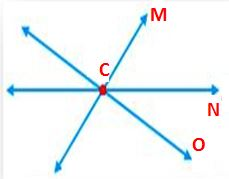From the above figure, The line M intersecting Lines N and O at a point c. But the Lines N and O are not parallel lines. Therefore, Line M is not a Transversal Line.

## Angles made by the Transversal with Two Lines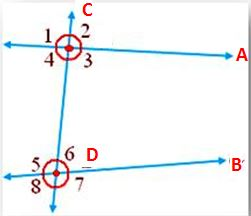From the above figure, Line C intersecting Line A and B (parallel lines). The angles formed around the Transversal line are called Transversal angles. From the given figure, ∠1, ∠2, ∠3, ∠4, ∠5, ∠6, ∠7, ∠8 are the angles with special names.

### Interior Angles

The angles that present in the area enclosed between two parallel lines and that are intersected by a transversal are also called interior angles. From figure, ∠3, ∠4, ∠5, ∠6 are interior angles.

### Exterior Angles

The angles that present outside two parallel lines and that are intersected by a transversal are called Exterior angles. From the figure, ∠1, ∠2, ∠7, ∠8 are Exterior angles.

### Corresponding Pair of Angles

The corresponding pair of angles present on the same side of the transversal. In the Pair of corresponding angles, if one is an interior angle, the other will be an exterior angle. Also, they do not form a linear pair. From the figure, (∠2, ∠6); (∠1, ∠5); (∠3, ∠7); (∠4, ∠8) are the Corresponding pair of angles.

### Pair of Alternate Angles

Pair of alternate angles present on the opposite sided of the transversal. Both Pair of Alternate Angles either interior angles or both are exterior angles. They will not form a linear pair. From the figure, (∠4, ∠6) and (∠3, ∠5) are interior alternate angles. Also, (∠1, ∠7) and (∠2, ∠8) are exterior alternate angles.

### Pair of Co-interior or Conjoined or Allied Angles

Pair of co-interior or Conjoined or Allied Angles are pairs of interior angles that present on the same side on the transversal. From the figure, (∠3, ∠6); (∠4, ∠5) are co-interior angles.

### Two Parallel Lines are cut by the TransversalWhen two parallel lines A and B are intersected by a Transversal line C, then

• the pairs of alternate angles are equal ∠4 = ∠6, ∠ 1 = ∠7, ∠3 =∠5, ∠2 = ∠8.
• the pairs of corresponding angles are equal ∠2 = ∠6, ∠1 = ∠5, ∠3 = ∠7, ∠4 = ∠8.
• Interior angles on the same side of transversal are supplementary ∠4 + ∠ 5 = 180°, ∠6 = 180°.

### Converse

When two parallel lines are intersected by a Transversal line

• pairs of alternate angles are equal
• pairs of corresponding angles are equal
• interior angles on the same side of the transversal are supplementary.

## Properties of Parallel Lines -Definition, Theorem & Examples

Parallel Lines are two lines in a plane, they do not intersect and parallel to each other. The distance between the parallel lines is always the same. The symbol to denote parallel lines is ||. Learn and practice all Lines and Angles concepts and problems on our website.From the above figure, Line A and Line B are parallel lines. We can write it as A || B and we can read it as A is parallel to B.

## Properties Of Angles Associated with Parallel Lines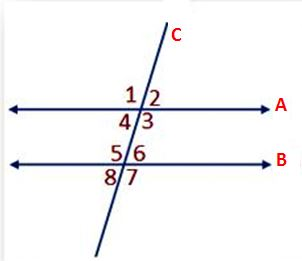From the above figure, A and B are two parallel lines and C is passing through two parallel lines by intersecting them at a point. C is a transversal line. If a line intersects two or more lines at distinct points then it is known as a transversal line. When two lines meet at a point in a plane, they are known as intersecting lines.

• The pair of corresponding angles is equal (∠4 = ∠8); (∠3 = ∠7); (∠2 = ∠6); (∠1 = ∠5).
• The pair of exterior alternate angles is equal (∠2 = ∠8); (∠1 = ∠7).
• The pair of interior alternate angles is equal (∠3 = ∠5); (∠4 = ∠6).
• Interior angles present on the same side of the transversal are supplementary, i.e., ∠4 + ∠5 = 180° and ∠3 + ∠6 = 180°.

Example:Look at the above figure and check out the parallel lines, transversal line, and etc.,
From the above figure, AB and CD are parallel lines and MN is a transversal line that cuts the parallel lines AB and CD.
(i) Interior and exterior alternate angles are equal.
i.e. ∠4 = ∠5 and ∠3 = ∠6 [Interior alternate angles]
∠2 = ∠7 and ∠1 = ∠8 [Exterior alternate angles]
(ii) Corresponding angles are equal.
i.e. ∠2 = ∠6; ∠1 = ∠5; ∠3 = ∠7 and ∠4 = ∠8
(iii) Co-interior or allied angles are supplementary.
i.e. ∠4 + ∠6 = 180° and ∠3 + ∠5 = 180°

### Conditions of Parallelism

Two straight lines are cut by a transversal, and if

• the two pairs of corresponding angles are equal and also the lines are parallel to each other.
• if the pair of interior angles on the same side of the transversal are supplementary, then the two straight lines are parallel.
• the pair of alternate angles are equal, then the straight lines are parallel to each other.

### Parallel Rays

Parallel Rays are two rays that do not intersect each other and have the same distance every time. A ray consists of one endpoint. If AB and CD are two rays and parallel rays, then it is represented as ray AB ∥ ray CD.

### Parallel Segments

If two line segments are parallel then those two line segments are called Parallel Segments. The line segment is a line that consists of two endpoints. If AB and CD are two line segments and parallel, then it is represented as line segment AB ∥ line segment CD.

## Vertically Opposite Angles – Definition, Theorem, Proof, Examples

Learn what are Vertically Opposite Angles and how to calculate them in this article. Vertically Opposite Angles are the angles formed when two lines intersect each other. The vertically opposite angles are always equal to each other. The adjacent angles of vertically opposite angles are always supplementary angles and they form 180º when we add them. Find Lines and Angles concepts and problems on our website.

If one angle is 35º, then the other angle is 180º – 35º.From the above figure, the line segment PQ and RS meet at the point O and these two lines are intersecting lines.

In a pair of intersecting lines, the angles which are opposite to each other form a pair of vertically opposite angles. From the figure, ∠SOQ and ∠POR form a pair of vertically opposite angles.

∠SOQ = ∠POR
∠SOP = ∠ROQ

Note: A vertical angle and its adjacent angle is supplementary to each other. It means they add up to 180 degrees.

## Vertical Angles Theorem and Proof

Theorem: In a pair of intersecting lines the vertically opposite angles are equal.

Proof: Consider two lines PQ and RS which intersect each other at O. The two pairs of vertical angles are:
i) ∠SOQ = ∠POR
ii) ∠SOP = ∠ROQIt can be seen that ray OS stands on the line PQ and according to Linear Pair Axiom, if a ray stands on a line, then the adjacent angles form a linear pair of angles.
Therefore, ∠SOQ + ∠SOP = 180° —(1) (Linear pair of angles)
Similarly, Ray OP stands on the line SR.
Therefore, ∠SOP + ∠POR = 180° —(2) (Linear pair of angles)
From (1) and (2),
∠SOQ + ∠SOP = ∠SOP + ∠POR
⇒ ∠SOQ = ∠POR —(3)
Also, OQ stands on the line SR.
Therefore, ∠SOQ + ∠QOR = 180° —(4) (Linear pair of angles)
From (1) and (4),
∠SOQ + ∠SOP = ∠SOQ + ∠QOR
⇒ ∠SOP = ∠QOR —(5)
Thus, the pair of opposite angles are equal.

Hence, proved.

### Vertically Opposite Angles Examples

1. In the given figure, find the measure of unknown angles.Solution:
From the given figure
(i) ∠C = 60° vertically opposite angles
(ii) ∠B = 90° vertically opposite angles
(iii) ∠B + ∠A + 60° = 180° (straight angle)
90° + ∠ A + 60° = 180°
150° + ∠ A = 180°
Therefore, ∠A = 180° – 150° = 30°
(iv) ∠A = ∠D vertically opposite angles

Therefore, ∠D = 30°

2. In the given figure, lines AB, CD, EF intersects at O. If M : N : P = 1 : 2 : 3, then find the values of M, N, P.Solution:
We know that the sum of all the angles at a point is 360°.
∠AOC = ∠DOB = M° (Pair of vertically opposite angles are equal.)
∠FOB = ∠AOE = N° (Pair of vertically opposite angles are equal.)
∠EOD = ∠COF = P° (Pair of vertically opposite angles are equal.)
Therefore, ∠AOE + ∠AOC + ∠COF + ∠FOB + ∠BOD + ∠DOE = 360°
N + M + P + N + M + P = 360°
⟹ 2M + 2N + 2P = 360°
⟹ 2(M + N + P) = 360°
⟹ M + N + P = 3̶6̶0̶°/2̶
⟹ M + N + P = 180° ——— (i)
Let the common ratio be a.
Therefore, M = a, N = 2a, P = 3a
Therefore, from the equation (i) we get;
a + 2a + 3a = 180°
⟹ 6a = 180°
⟹ a = 1̶8̶0̶°/6̶
⟹ a = 30°
Therefore, M = a, means M = 30°
N = 2a, means N = 2 × 30 = 60°
P = 3a, means P = 3 × 30 = 90°

Therefore, the measures of the angles are 30°, 60°, 90°.

## What is Linear Pair of Angles? | Axioms, Examples

Do you want to know What is linear pair of angles? Linear Pair of Angles are similar to adjacent angles but only the difference is adding two angles we get 180º in linear angles. Linear Pair of Angles will also have a common vertex, common arm like adjacent angles. Their interiors will also do not overlap. Remember that all adjacent angles may not form a linear pair. Learn all the Lines and Angles concepts in one place on our website.

## Properties of Linear Pair of Angles

Check the below Linear Pair of Angles properties listed below.

• They have a common vertex.
• They have a common arm.
• Also, they do not overlap.
• The sum of the angles is 180°.From the above figure, AO and OC are two opposite rays and ∠AOB and ∠BOC are the adjacent angles. Therefore, ∠AOB and ∠BOC form a linear pair.

### Explanation for Linear Pair of Angles

The angle between the two straight lines is 180° and they form a straight angle. The line segment is the portion of a line that consists of two endpoints. Also, a line with one endpoint is known as a ray. If you consider a line mn and the middle point is o. A ray OP dividing the two lines MO and ON. The complete angle MOP and angle PON becomes 180º to form a linear pair of angles.

∠MOP + ∠PON = ∠MON = 180°

We have also given Lines and Angles concepts and problems for free of cost on our website.

### Axioms

Axiom 1: If a ray stands on a line then the adjacent angles form a linear pair of angles.From the above figure, there are different line segments available that are passing through point O. From the figure, we can say that Angle BOD and Angle BOC are Linear Pair of Angles.

### Linear Pair of Angles Examples

In the given figure, ∠AOC and ∠ BOC form a linear pair if x – y = 50°, find the value of x and y.Solution:
Given x – y = 50° ………… (i)
We know that, x + y = 180° ………… (ii)